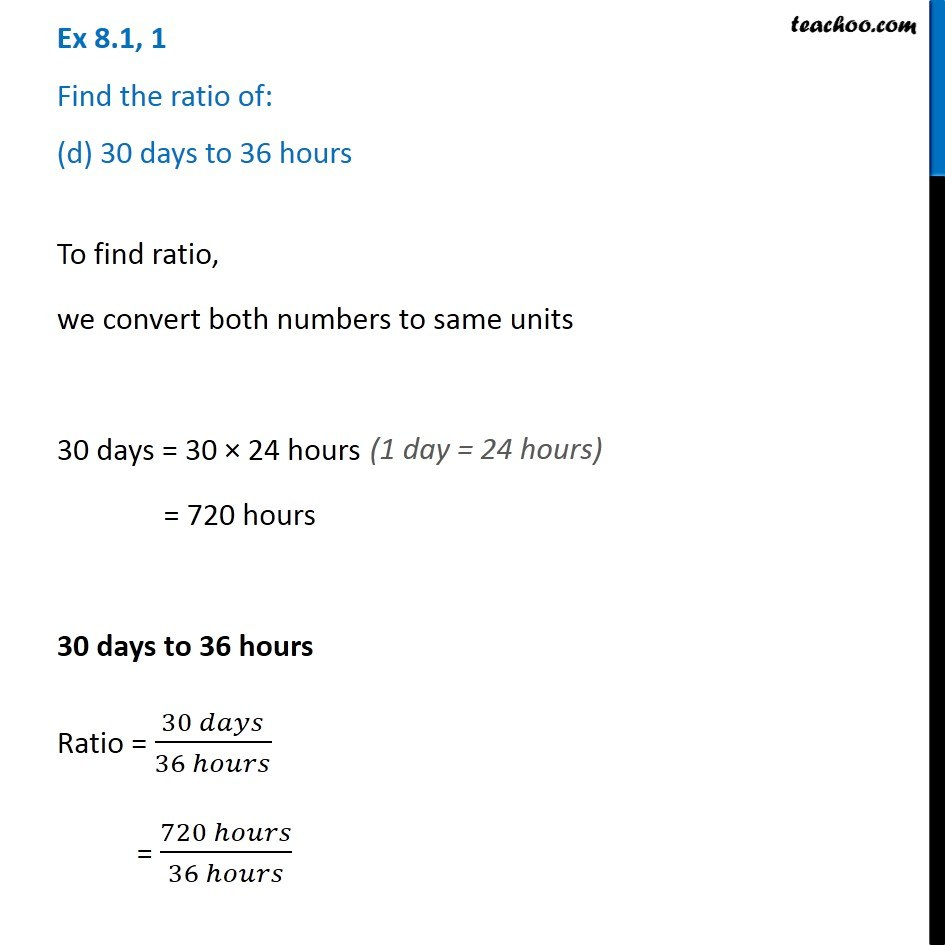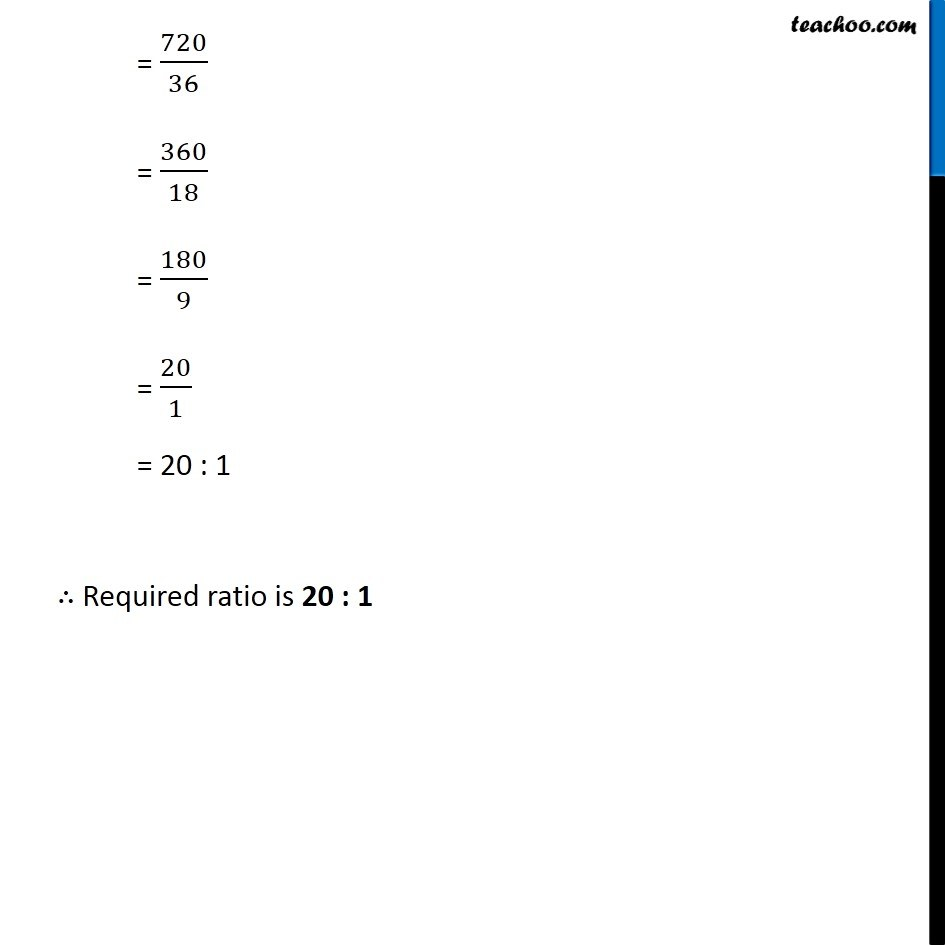Finding Ratios

Chapter 7 Class 7 Comparing Quantities
Serial order wiseLearn in your speed, with individual attention - Teachoo Maths 1-on-1 Class

### Transcript

Question 1 Find the ratio of: (d) 30 days to 36 hours To find ratio, we convert both numbers to same units 30 days = 30 × 24 hours = 720 hours 30 days to 36 hours Ratio = (30 𝑑𝑎𝑦𝑠)/(36 ℎ𝑜𝑢𝑟𝑠) = (720 ℎ𝑜𝑢𝑟𝑠)/(36 ℎ𝑜𝑢𝑟𝑠) (1 day = 24 hours) = 720/36 = 360/18 = 180/9 = 20/1 = 20 : 1 ∴ Required ratio is 20 : 1